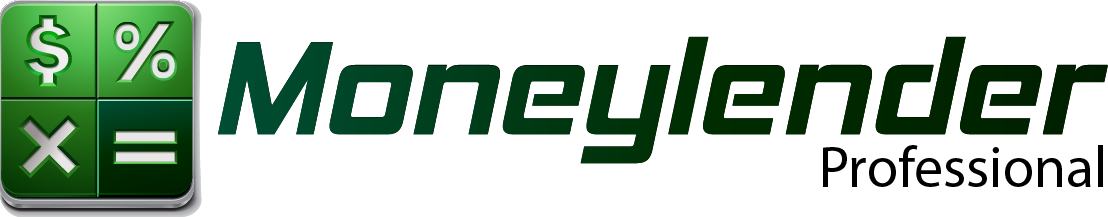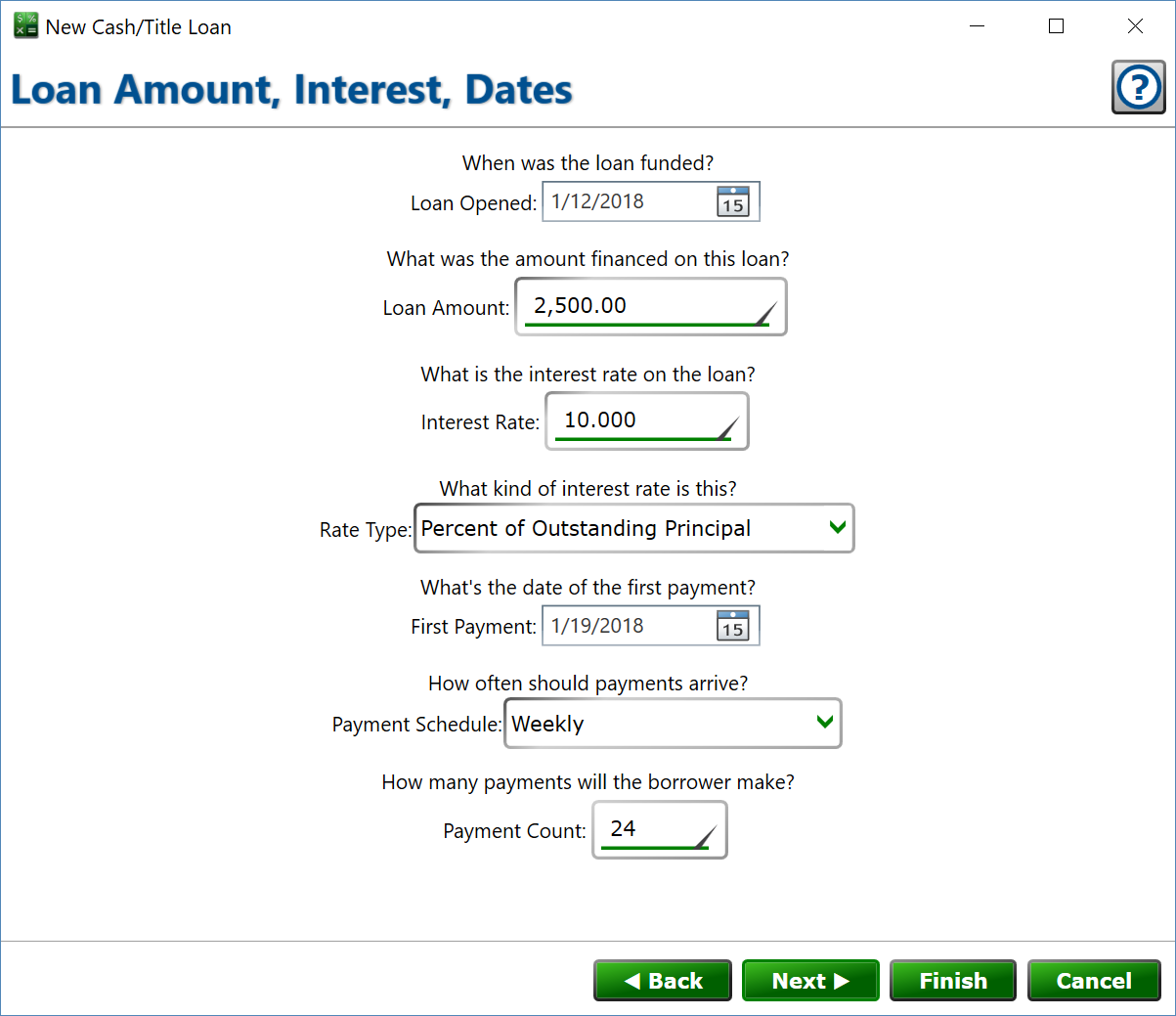# Loan Amount, Interest, DatesSet up the basic details of the cash loan here.

Loan Opened – enter the date when the loan was created and funds were disbursed to the borrower.

Loan Amount – enter the total amount finances for this loan. The will be the opening balance on the loan.

Interest Rate – used in conjunction with the rate type, this number will be used to determine the interest charges added to the loan.

Rate Type – choose the setting that matches how you want the interest calculated:

Annual Rate – The interest rate is an annual rate, so the interest will be adjusted by the amount of time that has gone by. This is how mortgages and car loans are calculated, and is commonly used in other loan types, too. If interest is monthly, for example, the balance will be multiplied by 1/12th of the rate set above to determine the interest earned for any given month.

Percent of Initial Principal – A percentage of the opening loan amount will be added every time interest is calculated, the interest added each cycle will remain the same over the life of the loan.

Percent of Outstanding Principal – The rate is a percentage of the loan's current balance. For example, if the rate is 10% and the loan has a balance of 1000 for this cycle, the interest will be 100. The amount of interest charged will decrease over the life of the loan as the borrower pays down the balance.

Fixed Currency Amount – The interest rate is a currency amount, and that number will be added every interest cycle. For example, an interest rate of 25 will add 25 to the loan each cycle – the balance is not a part of the calculation.

First Payment – enter the date when the first payment will be due on the loan.

Payment Schedule – how often should the borrower be making payments.

Payment Count – how many payments will the borrower make on this loan.